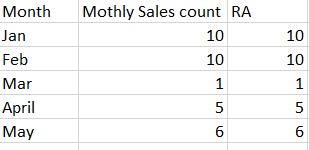cancel
Showing results for
Did you mean:New Member

## How to create rolling average from a measure

I have a measure: 'Monthly sales count'

This measure is calculated from `Monthly sales` table that has an established with `MyDate` table.

`MyDate` table has a column Month to retrieve month name from date.

Columns in `MyDate' table: `SalesDate` in mm/dd/yyyy formate, and `Month` with months name.

Here's the DAX i came up with to calulate 2 months of Rolling average:

RA =
VAR CurrentDate = LASTDATE('MyDate'[SalesDate])
VAR ThreeMonthsAgo = EDATE(CurrentDate, -3)
RETURN
AVERAGEX(
FILTER(
'MyDate',
'MyDate'[SalesDate] >= ThreeMonthsAgo && 'MyDate'[SalesDate] <= CurrentDate
),
CALCULATE([Monthly sales count])
)

Here's the result I get:How do I get the rolling average for each month?

Thank you!

1 ACCEPTED SOLUTIONNew Member

Solved:  CALCULATE([Measure],DATESINPERIOD( 'Date'[Date], MIN( 'Date'[Date] ) -1, -3, MONTH ))New Member

Solved:  CALCULATE([Measure],DATESINPERIOD( 'Date'[Date], MIN( 'Date'[Date] ) -1, -3, MONTH ))